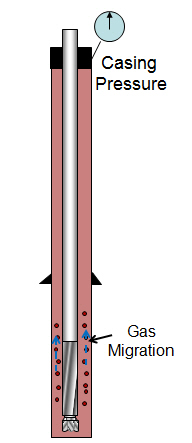## Determine the actual gas migration rate

You have learned how to estimate the gas migration rate. Today, I would like to show you how to figure out the actual gas migration rate after you shut the well in due to well control.The concept of this topic is to measure the pressure increment in a hour and divided by gradient of mud weight that you have in the well.

Let’s look at the formula:

Actual gas migration rate = Increase in casing pressure ÷ Pressure gradient of drilling mud

Where;

Actual gas migration rate in ft/hr

Increase in casing pressure in psi/hr

Pressure gradient of drilling mud in psi/ft

Let’s try to determine the actual gas migration rate with this following information:

Initial shut in casing pressure = 450 psi

Shut in casing pressure after half an hour shut in = 650 psi

Current mud weight = 12.5 ppg

Solution:

Pressure increase in half an hour = 650 – 450 = 200 psi

It means that pressure increase in an hour is 400 psi. Therefore, you get the increase in casing pressure = 400 psi/hr.

You need to calculate your mud weight in to ppg with this formula:

Mud gradient = 0.052 x mud weight

Mud gradient = 0.052 x 12.5 = 0.65 psi/ft